### AME 301: Differential Equations, Vibrations and Control Homework 6

 B. GoodwineJ. Lucey Fall, 2003 Issued: October 12, 2003 Due: October 15, 2003 (US)16 October 2003 (UK)

1. Consider the system in the following figure where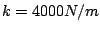,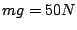,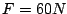and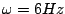.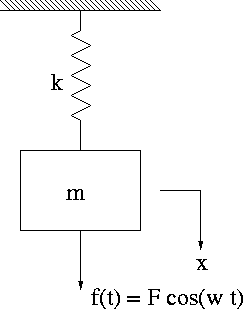Find

1. the extension of the spring due to the suspended weight;
2. the static displacement of the spring due to the maximum applied force; and,
3. the amplitude of the forced motion of the weight.

2. A spring-mass system like the one in the previous problem consists of a mass weighting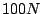and a spring with stiffness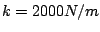. The mass is subjected to resonance by a harmonic force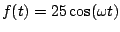. Find the amplitude of the forced motion at the end of
1.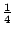cycle;
2.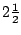cycles; and,
3.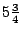cycles.

3. Considering the system in the previous figure, assume that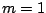,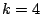,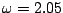and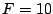. If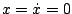, plot the response of the system for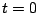to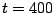. Explain the results.

4. Find the frequency ratio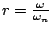at which the amplitude of a single degree of freedom damped system illustrated in the following figure attains the maximum value. Also find the value of the maximum amplitude.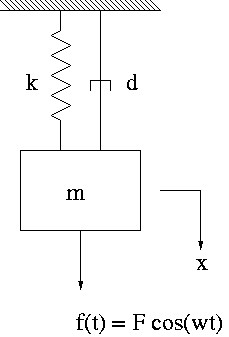5. For a vibrating system where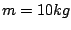,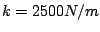and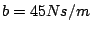, a harmonic force of amplitude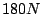and a frequency of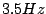acts on the mass. If the initial displacement and velocity of the mass are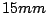and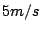, find the complete solution representing the motion of the mass.

6. An automobile is modeled as a single degree of freedom system vibrating in the vertical direction. It is driven along a road whose elevation varies sinusoidally. The distance from peak to trough is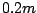and the distance along the road between the peaks is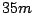. If the natural frequency of the automobile is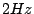and the dam;ping ratio of the shock absorbers is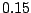, determine the amplitude of vibration of the automobile at a speed of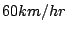. Also find the speed at which the ride would be most unfavorable for the passengers.

7. Consider the single degree of freedom building structure illustrated in the following figure.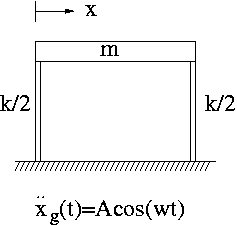Find the horizontal displacement of the floor (the mass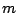) of the building frame where the ground acceleration is given by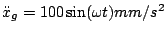. Let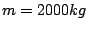,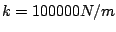,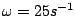and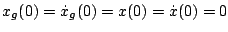.

8. A spring mass system is subjected to Coulomb damping. When a harmonic force of amplitude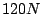and frequency of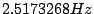is applied, the system is found to oscillate with an amplitude of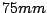. Determine the coefficient of dry friction ifand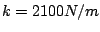.

Last updated: October 13, 2003.
B. Goodwine (goodwine@controls.ame.nd.edu)
J. Lucey (jlucey@nd.edu)